# Math in Focus Grade 7 Chapter 4 Lesson 4.4 Answer Key Solving Algebraic Inequalities

Practice the problems of Math in Focus Grade 7 Workbook Answer Key Chapter 4 Lesson 4.4 Solving Algebraic Inequalities to score better marks in the exam.

## Math in Focus Grade 7 Course 2 A Chapter 4 Lesson 4.4 Answer Key Solving Algebraic Inequalities

### Math in Focus Grade 7 Chapter 4 Lesson 4.4 Guided Practice Answer Key

Copy and complete. Solve each inequality and graph the solution set on a number line.

Question 1.
0.2x + 3 + 0.8x ≤ 4
0.2x + 3 + 0.8x ≤ 4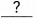+ 3 ≤ 4 Add the like terms.+ 3≤ 4 –Subtractfrom both sides.Simplify.
x ≤ 1

Explanation:
Given, 0.2x + 3 + 0.8x ≤ 4
x + 3 ≤ 4

Subtract 3 on both sides.

x + 3 – 3 ≤ 4 – 3

x ≤ 1

Question 2.
$$\frac{1}{4}$$x – 1 + $$\frac{3}{4}$$x > 0
$$\frac{1}{4}$$x – 1 + $$\frac{3}{4}$$x > 0– 1 > 0 Add the like terms.– 1 +> 0 +Addto both sides.>Simplify.
x > 1

Explanation:
Given, (1/4)x – 1 + (3/4)x >0

x – 1 > 0

x – 1 + 1 > 0 + 1

x > 1

Solve each inequality and graph the solution set on a number line.

Question 3.
2x + 3 < 13 + x
x < 10

Explanation:
Given, 2x + 3 < 13 + x

Subtract x and 3 on both sides.

2x + 3 – x – 3 < 13 + x – x – 3

x < 10

Question 4.
1.5x – 3 ≥ 4 + 0.5x
x ≥ 7

Explanation:
Given, 1.5x – 3 ≥ 4 + 0.5x

Subtract 0.5x o both sides.

1.5x – 3 – 0.5x ≥ 4 + 0.5x – 0.5x

1x – 3 ≥ 4

x – 3 + 3 ≥ 4 + 3

x ≥ 7

Question 5.
4 + $$\frac{1}{3}$$x > 8 + $$\frac{4}{3}$$x
x < 4

Explanation:
Given, 4 + (1/3)x > 8 + (4/3)x

Subtract (1/3)x on both sides.

4 + (1/3)x – (1/3)x > 8 + (4/3)x – (1/3)x

4 > 8 -x

Subtract 8 on both sides.

4 – 8 > 8 -x – 8

-x < -4

x < 4

Hands-On Activity

EXPLORE DIVISION AND MULTIPLICATIVE PROPERTIES OF AN INEQUALITY

Work individually.

Step 1: Use a copy of this table. Complete each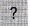using the symbols > or <.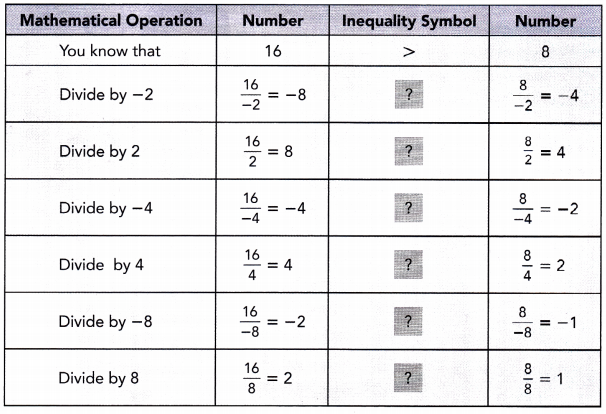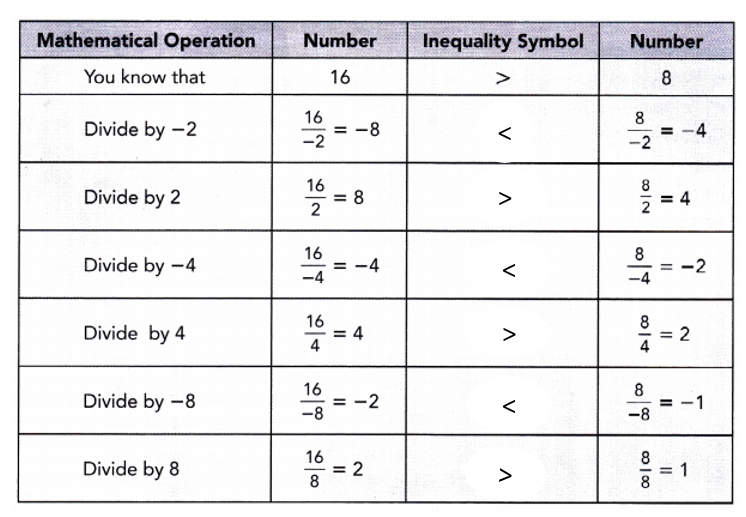Math Journal What happens to the direction of the inequality symbol when you divide by a positive number? Based on your observation, write a rule for dividing both sides of an inequality by a positive number.

Math Journal What happens to the direction of the inequality symbol when you divide by a negative number? Based on your observation, write a rule for dividing both sides of an inequality by a negative number.

Step 2: Use a copy of this table. Complete eachusing the symbols > or <.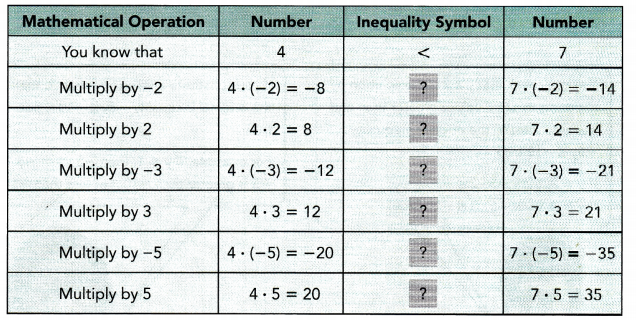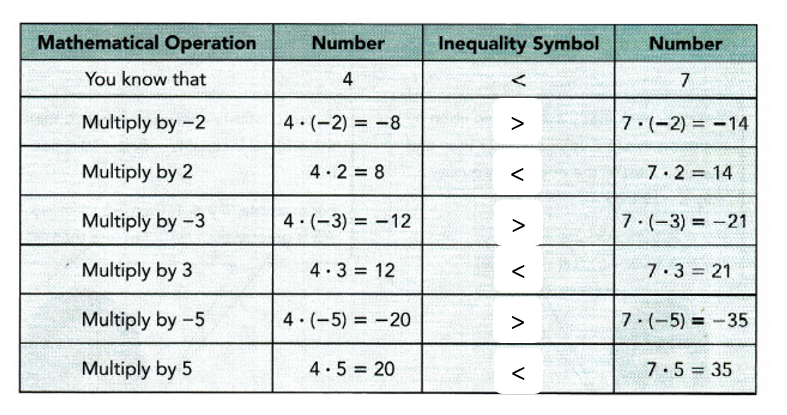Math Journal What happens to the direction of the inequality symbol when you multiply by a positive number? Based on your observation, write a rule for multiplying both sides of an inequality by a positive number.

Math Journal What happens to the direction of the inequality symbol when you multiply by a negative number? Based on your observation, write a rule for multiplying both sides of an inequality by a negative number.

Solve each inequality and graph the solution set on a number line.

Question 6.
–$$\frac{1}{5}$$w ≤ 2
x ≥ -10

Explanation:
Given, -(1/5)x ≤ 2

Multiply 5 on both sides

-(1/5)x × 5  ≤ 2 × 5

-x ≤ 10

x ≥ -10

Question 7.
-7m > 21
m < -3

Explanation:
Given, -7m > 21

Divide 7 on both sides.

-7m ÷ 7 > 21 ÷ 7

-m > 3

m < -3

Question 8.
6 > -0.3y
y > -20

Explanation:
Given, 6 > -0.3y

Divide 0.3 on both sides.

6 ÷ 0.3 > -0.3y ÷ 0.3

20 > -y

y > -20

Solve each inequality and graph the solution set on a number line.

Question 9.
4y + 7 < 27
4y + 7 < 27
4y + 7 –< 27 –Subtractfrom both sides.
4y <Simplify.
4y ÷<÷Divide both sides by.
y <Simplify.
y < 5

Explanation:
Given,  4y + 7 < 27

Subtract 7 on  both sides.

4y + 7 – 7 < 27 – 7

4y < 20

Divide 4 on both sides

4y ÷ 4 < 20 ÷ 4

y < 5

Question 10.
-5y – 9 > 21
-5y – 9 > 21
-5y – 9 +≥ 21 +Addto both sides.
-5y ≥Simplify.
– 5y ÷÷Divide both sides byand reverse the inequality symbol.
y ≤Simplify.
y ≤ -6

Explanation:
Given, -5y – 9 > 21

-5y – 9 + 9 > 21 + 9

– 5y > 30

Divide 5 on both sides.

– 5y ÷ 5 > 30 ÷ 5

-y < 6

y ≤-6

Question 11.
$$\frac{1}{2}$$x + $$\frac{3}{4}$$ ≥ 5
x ≥  (17/2)

Explanation:
Given, (1/2)x  + (3/4) ≥ 5

Subtract (3/4) on both sides.

(1/2)x  + (3/4) – (3/4) ≥ 5 – (3/4)

(1/2)x ≥  (20 – 3)/4

(1/2)x ≥  (17/4)

Multiply 2 on both sides

(1/2)x × 2 ≥  (17/4) × 2

x ≥  (17/2)

Question 12.
1.5 – 0.3y > 3.6
y < -7

Explanation:
Given, 1.5 – 0.3y > 3.6

Subtract 1.5 on both sides.

1.5 – 0.3y – 1.5 > 3.6 – 1.5

-0.3y > 2.1

Divide -0.3 on both sides

-0.3y ÷ (-0.3) > 2.1 ÷ (-0.3)

y < -7

Question 13.
-8y + 32 ≤ -17 – y
y ≥ 7

Explanation:
Given, -8y + 32 ≤ -17 – y

-8y + 32 + y ≤ -17 – y + y

-7y +32 ≤ -17

Subtract 32 on both sides.

-7y +32 – 32 ≤ -17 -32

-7y ≤ -49

Divide -7 on both sides.

-7y ÷ (-7) ≤ -49 ÷ (-7)

y ≥ 7

Question 14.
4(2 – y) ≥ 20
y ≤ -3

Explanation:
Given, 4(2 – y) ≥ 20

8 – 4y ≥ 20

Subtract  8 on both sides

8 – 4y – 8 ≥ 20 – 8

-4y ≥ 12

Divide -4 on both sides

-4y ÷ (-4) ≥ 12 ÷ (-4)

y ≤ -3

### Math in Focus Course 2A Practice 4.4 Answer Key

Solve each inequality using addition and subtraction. Then graph each solution set on a number line.

Question 1.
x + 8 > 14
x > 6

Explanation:
Given, x + 8 > 14

Subtract 8 on both sides.

x + 8 – 8 > 14 – 8

x > 6

Question 2.
2 ≥ x – 12
x ≤14

Explanation:
Given, 2 ≥ x – 12

2 + 12 ≥ x – 12 + 12

14 ≥ x

x ≤14

Question 3.
-7x + 5 + 8x > 3
x > -2

Explanation:
Given, -7x + 5 + 8x > 3

x + 5 > 3

Subtract 5 on both sides.

x + 5 – 5 > 3 – 5

x > -2

Question 4.
– 2x – 3 + 3x ≥ 12
x ≥ 15

Explanation:
Given, -2x – 3 + 3x ≥ 12

x – 3 ≥ 12

x – 3 + 3 ≥ 12 + 3

x ≥ 15

Question 5.
29 < $$\frac{2}{3}$$x + 14 + $$\frac{1}{3}$$x
x>15

Explanation:
29 < (2/3)x + 14 + (1/3)x

Subtract 14 on both sides.

29 – 14 < (2/3)x + 14 + (1/3)x – 14

15 < x

x>15

Question 6.
$$\frac{1}{5}$$x + 9 + $$\frac{4}{5}$$x > -11
x > -20

Explanation:
Given, (1/5)x + 9 + (4/5)x > -11

Subtract 9 on both sides.

(1/5)x + 9 + (4/5)x – 9 > -11 – 9

x > -20

Question 7.
0.7x + 4 + 0.3x ≤ 10
x ≤ 6

Explanation:
Given, 0.7x + 4 + 0.3x ≤ 10

Subtract 4 on both sides.

0.7x + 4 + 0.3x – 4 ≤ 10 – 4

x ≤ 6

Question 8.
0.4x – 6 + 0.6x ≥ 19
x ≥ 25

Explanation:
Given , 0.4x – 6 + 0.6x ≥ 19

x – 6 ≥ 19

x – 6 + 6 ≥ 19 + 6

x ≥ 25

Question 9.
3x + 4 < 2x + 9
x < 5

Explanation:
Given, 3x + 4 < 2x + 9

Subtract 4 on both sides.

3x + 4 – 4 < 2x + 9 – 4

3x < 2x + 5

Subtract 2x on both sides

3x – 2x < 2x + 5 – 2x

x < 5

Question 10.
8 – 4x > 12 – 3x
x < -4

Explanation:
Given, 8 – 4x > 12 – 3x

8 – 4x + 3x > 12 – 3x +3x

8 -x > 12

Subtract 8 on both sides.

8 -x – 8 > 12 – 8

-x > 4

x < -4

Question 11.
$$\frac{2}{3}$$x + 2 ≥ 9 – $$\frac{1}{3}$$x
x ≥ 7

Explanation:
Given, (2/3)x + 2 ≥ 9 – (1/3)x

(2/3)x + 2 + (1/3)x ≥ 9 – (1/3)x + (1/3)x

x +2 ≥ 9

Subtract 2 on both sides.

x +2 – 2 ≥ 9 – 2

x ≥ 7

Question 12.
13 + 1$$\frac{3}{5}$$x ≥ 18 + $$\frac{3}{5}$$x
x ≥ 5

Explanation:
Given, 13 + 1(3/5)x ≥ 18 + (3/5)x

13 + (8/5)x ≥ 18 + (3/5)x

Subtract (3/5)x on both sides.

13 + (8/5)x – (3/5)x ≥ 18 + (3/5)x – (3/5)x

13 + x ≥ 18

Subtract 13 on both sides.

13 + x – 13 ≥ 18 – 13

x ≥ 5

Question 13.
1.7x + 5 < 16 + 0.7x
x < 11

Explanation:
Given, 1.7x + 5 < 16 + 0.7x

Subtract 0.7x on both sides.

1.7x + 5 – 0.7x < 16 + 0.7x – 0.7x

5  + x < 16

Subtract 5 on both sides.

5  + x – 5 < 16 – 5

x < 11

Question 14.
8.5 – 0.9x > 9.8 – 1.9x
x < 1.3

Explanation:
Given, 8.5 – 0.9x > 9.8 – 1.9x

8.5 – 0.9x + 0.9x > 9.8 – 1.9x + 0.9x

8.5 > 9.8 – x

Subtract 9.8 on both sides.

8.5  – 9.8 > 9.8 – x – 9.8

-1 .3 > – x

x < 1.3

Question 15.
Math Journal Solve the inequality 8 + 2x ≥ 12 and show your work. What value is a solution of 8 + 2x ≥ 12 but is not a solution of 8 + 2x > 12?
x ≥ 2, x > 2 Both  the solutions are not same.

Explanation:
Given, two equations: 8 + 2x ≥ 12

8 + 2x > 12

Let us solve 1st equation: 8 + 2x ≥ 12

Subtract 8 on both sides.

8 + 2x – 8 ≥ 12 – 8

2x ≥ 4

Divide 2 on both sides

2x ÷ 2 ≥ 4 ÷ 2

x ≥ 2

Let us solve 2nd equation:
8 + 2x > 12

Subtract 8 on both sides.

8 + 2x – 8 > 12 – 8

2x > 4

Divide 2 on both sides

2x ÷ 2 > 4 ÷ 2

x > 2

Question 16.
Math Journal Eric solved the inequality 6y ≤ -18 as shown below:
6y ≤ -18
6y ÷ 6 ≥ -18 ÷ 6
y ≥ -3
Describe and correct the error that Eric made.
y ≥ 3

Explanation:
Given, 6y ≤ -18

Divide 6 on both sides.

6y ÷ 6 ≥ -18 ÷ 6

y ≥ 3

Solve each inequality using division and multiplication. Then graph the solution set on a number line.

Question 17.
3 ≥ -3x
x ≥ -1

Explanation:
Given, 3 ≥ -3x

Divide 3 on both sides.

3 ÷ 3 ≥ -3x ÷ 3

1 ≥ -x

x ≥ -1

Question 18.
-4x > 12
x < -3

Explanation:
Given, -4x > 12

Divide -4 on both sides.

-4x ÷ – 4 > 12 ÷ – 4

x < -3

Question 19.
–$$\frac{x}{5}$$ ≤ 2
x ≥ -10

Explanation:
Given, -(x/5) ≤ 2

Multiply -5 on both sides.

-(x/5)  ≤ 2

-(x/5) × -5 ≤ 2 × -5

x ≥ -10

Question 20.
–$$\frac{2}{3}$$x > 8
x < -12

Explanation:
Given, -(2/3)x > 8

Multiply 3 on both sides.

-(2/3)x × 3 > 8 × 3

-2x > 24

Divide -2 on both sides.

-2x ÷ -2 > 24 ÷ -2

x < -12

Question 21.
-0.2x ≥ 6
x ≤ -30

Explanation:
Given, -0.2x ≥ 6

Divide -0.2 on both sides.

-0.2x ÷ -0.2 ≥ 6 ÷ -0.2

x ≤ -30

Question 22.
9 > -0.5x
x > – 18

Explanation:
Given, 9 > -0.5x

Divide – 0.5 on both sides.

9 ÷ -0.5 > -0.5x ÷ -0.5

-18 >x

x > – 18

Solve each inequality using the four operations. Then graph each solution set on a number line.

Question 23.
7y – 3 > 11
y > 2

Explanation:
Given, 7y – 3 > 11

7y – 3 + 3 > 11 + 3

7y > 14

Divide 7 on both sides.

7y ÷ 7 > 14 ÷ 7

y > 2

Question 24.
-3a + 5 < -7
a > 4

Explanation:
Given, -3a + 5 < -7

Subtract 5 on both sides.

-3a + 5 – 5 < -7 – 5

-3a < -12

Divide -3 on both sides.

-3a ÷ -3 < -12 ÷ -3

a > 4

Question 25.
$$\frac{x}{4}$$ + $$\frac{3}{16}$$ ≥ 1
x ≥ (13/4)

Explanation:
(x/4) + (3/16) ≥ 1

multiply 4 on both sides.

((x/4) + (3/16) ) ×  4 ≥ 1 ×  4

x + (3/4) ≥ 4

Subtract (3/4) on both sides.

x + (3/4) – (3/4) ≥ 4 –  (3/4)

x ≥ (13/4)

Question 26.
$$\frac{3}{5}$$a – $$\frac{4}{5}$$ < $$\frac{7}{10}$$
a < (5/2)

Explanation:
Given, (3/5)a – (4/5) < (7/10)

(3/5)a – (4/5) + (4/5) < (7/10) + (4/5)

(3/5)a < ((7+(4 × 2))/10)

(3/5)a < (7+8)/10

(3/5)a < 15/10

Divide 3 on both sides.

(3/5)a ÷ 3 < 15/10 ÷ 3

(a/5) < (5/10)

(a/5) < (1/2)

Multiply 5 on both sides.

(a/5) × 5 < (1/2) × 5

a < (5/2)

Question 27.

7 – 0.3x > 4
x < 10

Explanation:
Given, 7 – 0.3x > 4

Subtract 7 on both sides.

7 – 0.3x – 7 > 4 – 7

-0.3x > -3

Divide -0.3 on both sides.

-0.3x ÷ -0.3 > -3 ÷ -0.3

x < 10

Question 28.
2.4y + 5 < 29
y < 10

Explanation:
Given, 2.4y + 5 < 29

Subtract 5 on both sides.

2.4y + 5 – 5 < 29 – 5

2.4y < 24

Divide 2.4 on both sidea.

2.4y ÷ 2.4 < 24 ÷ 2.4

y < 10

Question 29.
5x + 3 < 7 + 7x
x > -2

Explanation:
Given, 5x + 3 < 7 + 7x

Subtract 3 on both sides.

5x + 3 – 3 < 7 + 7x – 3

5x < 4 + 7x

subtract 5x on both sides.

5x -5x < 4 + 7x – 5x

0 < 4 + 2x

-4 < 2x

2x > -4

Divide 2 on both sides.

2x ÷ 2 > -4 ÷ 2

x > -2

Question 30.
11 – 7x ≤ 20 – 8x
x ≤ 9

Explanation:
Given, 11 – 7x ≤ 20 – 8x

Subtract 11 on both sides.

11 – 7x – 11 ≤ 20 – 8x – 11

-7x ≤ 9 – 8x

8x – 7x ≤ 9

x ≤ 9

Question 31.
$$\frac{4}{3}$$ – $$\frac{5}{6}$$x ≥ –$$\frac{1}{6}$$ – $$\frac{2}{3}$$x
x ≤ 7

Explanation:
Given, (4/3) – (5/6)x ≥ -(1/6) – (2/3)x

(4/3) – (5/6)x + (2/3)x ≥ -(1/6) – (2/3)x + (2/3)x

(4/3) – ((5x – 4x)/6) ≥ -(1/6)

(4/3) -(x/6) ≥ -(1/6)

(4/3) -(x/6) + (1/6) ≥ -(1/6) + (1/6)

((6 + 1)/6) – x/6 ≥  0

(7/6) – x/6 ≥  0

7 – x ≥  0

7 ≥  x

x ≤ 7

Question 32.
$$\frac{2}{5}$$x + 4 ≤ $$\frac{7}{10}$$x – 8
x ≥ 40

Explanation:
Given, (2/5)x + 4 ≤ (7/10)x – 8

Subtract 4 on both sides.

(2/5)x + 4 – 4 ≤ (7/10)x – 8 – 4

(2/5)x ≤ (7/10)x – 12

12 ≤ (7/10)x  – (2/5)x

12 ≤  (7 – 4)x/10

12 ≤ 3x/10

Multiply 10 on both sides.

12 × 10 ≤ 3x/10 × 10

120 ≤ 3x

Divide 3 on both sides.

120 ÷ 3 ≤ 3x ÷ 3

40 ≤ x

x ≥ 40

Question 33.
5.4x + 4.2 – 3.8x > 9
x > 3

Explanation:
Given, 5.4x + 4.2 – 3.8x > 9

1.6x + 4.2 > 9

Subtract 4.2 on both sides.

1.6x + 4.2 – 4.2 > 9 – 4.2

1.6x > 4.8

Divide 1.6 on both sides.

1.6x > 4.8

1.6x ÷ 1.6 > 4.8 ÷ 1.6

x > 3

Question 34.
6.6 + 1.3x – 5.2x ≤ 14

Explanation:
Given, 6.6 + 1.3x – 5.2x ≤ 14

Subtract 6.6 on both sides.

6.6 + 1.3x – 5.2x – 6.6 ≤ 14 – 6.6

-3.9x ≤ 7.4

Divide -3.9 on both sides.

-3.9x ÷ -3.9 ≤ 7.4 ÷ -3.9

x ≥ -(74/39)

Solve each inequality with parentheses using the four operations.

Question 35.
3(y + 2) ≤ 18
y ≤ 4

Explanation:
Given, 3(y + 2) ≤ 18
Divide 3 on the both sides.

3(y + 2) ÷ 3 ≤ 18 ÷ 3

(y + 2) ≤ 6

Subtract 2 on both sides.

y + 2 – 2 ≤ 6 – 2

y ≤ 4

Question 36.
8(y – 1) > 24
y > 4

Explanation:
Given, 8(y – 1) > 24

Divide 8 on both sides.

8(y – 1) ÷ 8 > 24 ÷ 8

y – 1 > 3

y – 1 + 1 > 3 + 1

y > 4

Question 37.
$$\frac{1}{2}$$(a + 1) ≤ 4
a ≤ 7

Explanation:
Given, (1/2) (a + 1) ≤ 4

Multiply 2 on both sides.

(1/2) (a + 1) × 2 ≤ 4 × 2

a +1 ≤ 8

Subtract 1 on both sides.

a +1 -1 ≤ 8 – 1

a ≤ 7

Question 38.
$$\frac{2}{3}$$(3 – a) < 3
a > -(3/2)

Explanation:
Given, (2/3)(3 – a) < 3

Divide (2/3) on both sides.

(2/3)(3 – a) ÷ (2/3) < 3 ÷ (2/3)

(3 – a) < (9/2)

Subtract  3 on both sides.

3 – a – 3 < (9/2) – 3

-a  < (9-6)/2

a > -(3/2)

Question 39.
1.3(2 – x) > 3.9
x < -1

Explanation:
Given, 1.3(2 – x) > 3.9

Divide 1.3 on both sides.

1.3(2 – x) ÷ 1.3 > 3.9 ÷ 1.3

2 – x > 3

Subtract 2 on both sides.

2 – x – 2 > 3 – 2

-x > 1

x < -1

Question 40.
3.6(5x – 1) < 5.4
x < (1/2)

Explanation:
Given, 3.6(5x – 1) < 5.4

Divide 3.6 on both sides.

3.6(5x – 1) ÷ 3.6 < 5.4 ÷ 3.6

5x -1 < 1.5

5x -1 + 1 < 1.5 + 1

5x < 2.5

Divide 5 on both sides.

5x ÷ 5 < 2.5 ÷ 5

x < (1/2)

Question 41.
4 + 2(1 – 3y) < 36
y > -5

Explanation:
Given, 4 + 2(1 – 3y) < 36

Subtract 4 on both sides.

4 + 2(1 – 3y) – 4 < 36 – 4

2(1 – 3y) < 32

Divide 2 on both sides.

2(1 – 3y) ÷ 2 < 32 ÷ 2

1 – 3y < 16

Subtract 1 on both sides.

1 – 3y – 1 < 16 – 1

-3y < 15

Divide -3 on both sides.

-3y ÷ -3 < 15 ÷ -3

y > -5

Question 42.
2(3 – x) > 5x – 1
x < 1

Explanation:
Given, 2(3 – x) > 5x – 1

6 – 2x > 5x – 1

6 – 2x + 1 > 5x – 1 + 1

7 -2x > 5x

7 -2x + 2x > 5x + 2x

7 > 7x

Divide 7 on both sides.

7 ÷ 7 > 7x ÷ 7

1 > x

x < 1

Question 43.
$$\frac{5}{9}$$(x + 1) ≥ $$\frac{2}{3}$$
x ≥ (1/5)

Explanation:
Given, (5/9)(x + 1) ≥ (2/3)

Multiply 9 on both sides.

(5/9)(x + 1) × 9 ≥ (2/3) × 9

5 (x + 1) ≥  2 × 3

5 (x + 1) ≥ 6

Divide 5 on both sides.

5 (x + 1) ÷ 5  ≥ 6 ÷ 5

x + 1 ≥ (6/5)

Subtract 1 on both sides.

x + 1 – 1 ≥ (6/5) – 1

x ≥ (6-5)/5

x ≥ (1/5)

Question 44.
$$\frac{2}{3}$$(1 – 3x) > $$\frac{1}{6}$$
x < (1/4)

Explanation:
Given,  (2/3) (1 – 3x) > (1/6)

Multiply 3 on both sides.

(2/3) (1 – 3x) × 3 > (1/6) × 3

2 (1 – 3x) > (1/2)

Divide 2 on both sides.

2 (1 – 3x) ÷ 2 > (1/2) ÷ 2

1 – 3x > (1/4)

Subtract 1 on both sides.

1 – 3x – 1 > (1/4) – 1

-3x > -(3/4)

Divide  -3 on both sides.

-3x ÷ -3 > -(3/4) ÷ -3

x < (1/4)

Question 45.
1.7 + 0.2(1 – x) ≥ 2.7
x ≤ -4

Explanation:
Given, 1.7 + 0.2(1 – x) ≥ 2.7

1.7 + 0.2 -0.2x ≥ 2.7

1.9 – 0.2x ≥ 2.7

Subtract 1.9 on both sides.

1.9 – 0.2x – 1.9 ≥ 2.7 – 1.9

-0.2x ≥ 0.8

Divide -0.2 on both sides.

-0.2x ÷ -0.2 ≥ 0.8 ÷ -0.2

x ≤ -4

Question 46.
2.5(3 – 2x) + 1 ≥ 29
x ≤ 4.1

Explanation:
Given, 2.5(3 – 2x) + 1 ≥ 29

7.5 – 5x + 1 ≥ 29

8.5 -5x ≥ 29

Subtract 8.5 on both sides.

8.5 -5x – 8.5 ≥ 29 – 8.5

-5x ≥ 20.5

Divide -5 on both sides.

-5x ÷ -5  ≥ 20.5 ÷ -5

x ≤ 4.1

Question 47.
Math Journal Compare solving the inequality -5(x + 6) < 10 with solving the equation -5(x + 6) = 10. Describe the similarities and differences between solving the inequality and solving the equation. Explain how the solution set of the inequality -5(x + 6) < 10 is different from the solution of the equation -5(x + 6) = 10.
The solution of the equation -5(x + 6) < 10 is  x > -8, which represents the number x can be any number greater than -8.

The solution of the equation -5(x + 6) = 10 is x = -8, here the number x will be only -8 not any other number.

Explanation:
Given two equations are, -5(x + 6) < 10 and  -5(x + 6) = 10

Let us consider -5(x + 6) < 10

Divide -5 on both sides.

-5(x + 6) ÷ -5 < 10 ÷ -5

(x + 6) > -2

Subtract 6 on both sides.

x + 6 – 6 > -2 – 6

x > -8

Let us consider the second equation -5(x + 6) = 10

Divide -5 on both sides.

-5(x + 6) ÷ -5 = 10 ÷ -5

(x + 6) = -2

Subtract 6 on both sides.

x + 6 – 6 = -2 – 6

x = -8

Solve each inequality using the four operations.

Question 48.
10 – 3(4a – 3) < 2(3a – 4) – 9.2
a > 2.01

Explanation:
Given, 10 – 3(4a – 3) < 2(3a – 4) – 9.2

10 – 12a + 9 < 6a – 8 – 9.2

19 – 12a < 6a – 17.2

Subtract  6a on both sides.

19 – 12a – 6a < 6a – 17.2 – 6a

19 – 18a < -17.2

Subtract 19 on both sides.

19 – 18a – 19 < -17.2 – 19

-18a <-36.2

Divide -18 on both sides.

-18a ÷ -18 <-36.2 ÷ -18

a > 2.01

Question 49.

7(2a – 3) ≤ 5 – 2(3a – 1)
a ≤  (7/5)

Explanation:
Given, 7(2a – 3) ≤ 5 – 2(3a – 1)

14a – 21 ≤ 5 – 6a + 2

14a -21 ≤ 7 – 6a

Subtract 7 on both sides.

14a -21 – 7 ≤ 7 – 6a – 7

14a -28 ≤ -6a